# Frank Solutions for Class 10 Maths Chapter 2 Sales Tax and Value Added Tax

Frank Solutions for Class 10 Maths Chapter 2 Sales Tax and Value Added Tax, are provided here. BYJU’S tutor team has prepared solutions which help students attain good marks in Maths. From the exam point of view, the solutions are solved in a simple manner where students can secure an excellent score by solving the Frank Solutions for Class 10 Maths Chapter 2. Solutions that are provided here will help you in getting acquainted with a wide variety of questions and thus, develop problem-solving skills.

Chapter 2 – Sales Tax and Value Added Tax, Sales tax is an amount of money, calculated as a percentage that is added to the cost of a product or service when purchased by a consumer at a retail location. Value Added Tax is paid at each stage in the production of goods or services and by the final customer.

## Download the PDF of Frank Solutions for Class 10 Maths Chapter 2 Sales Tax and Value Added Tax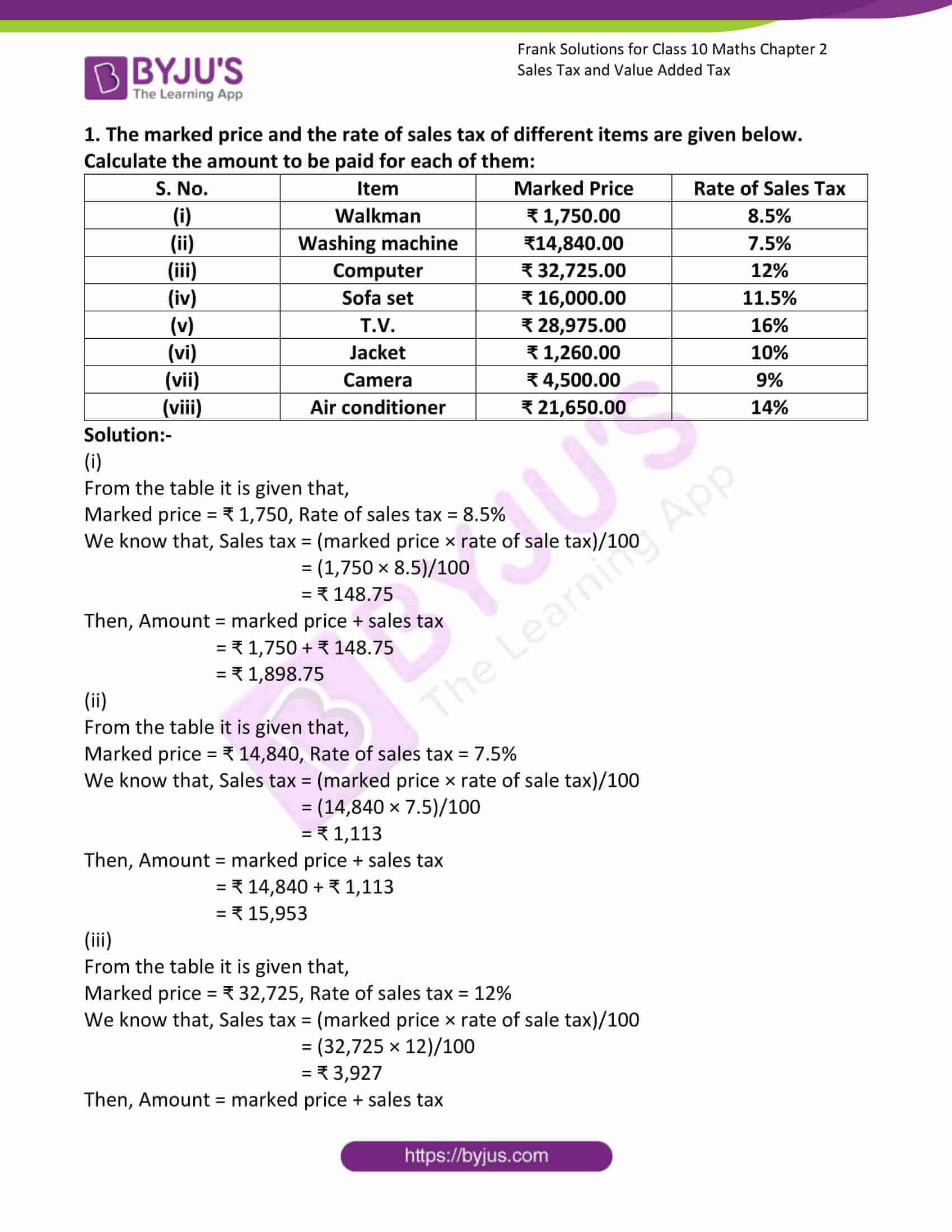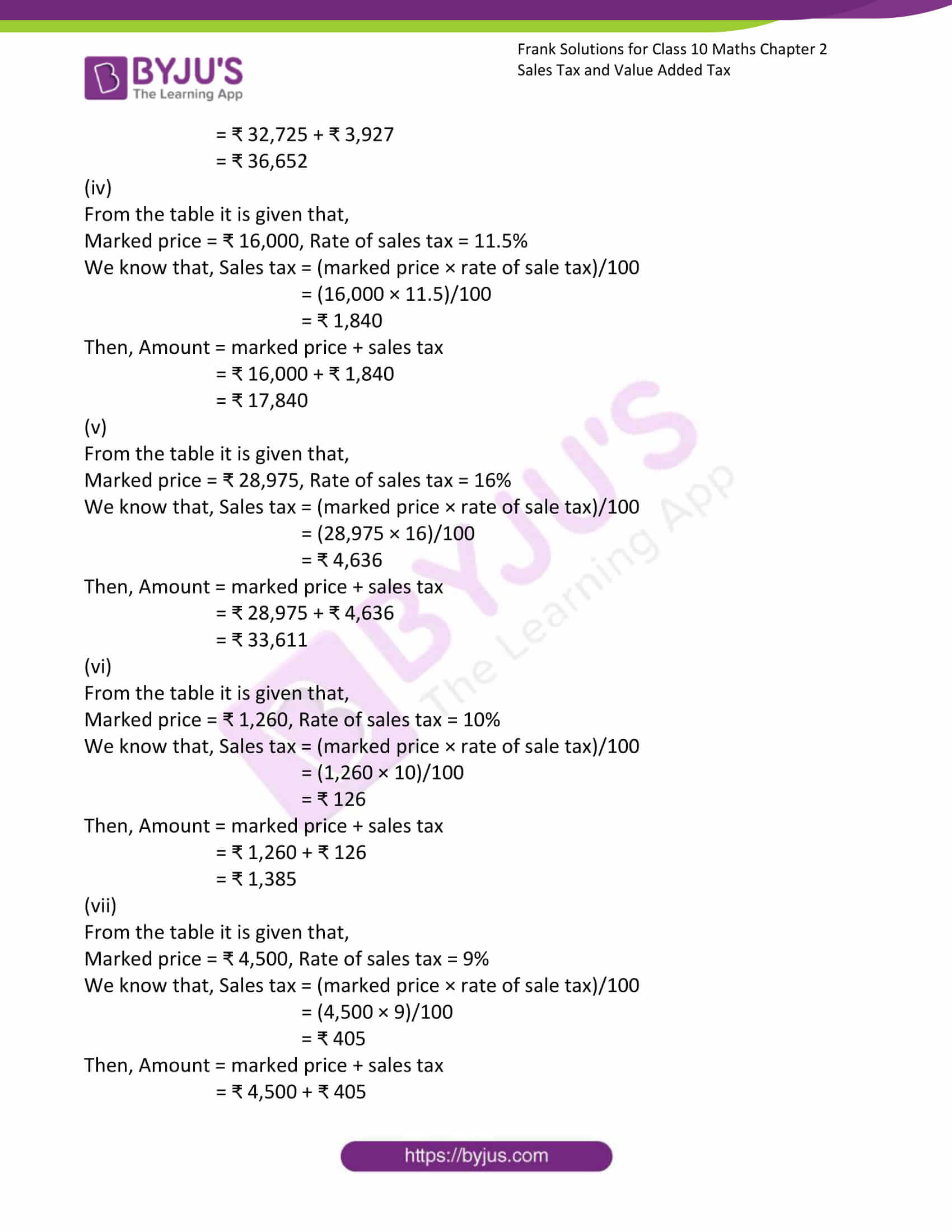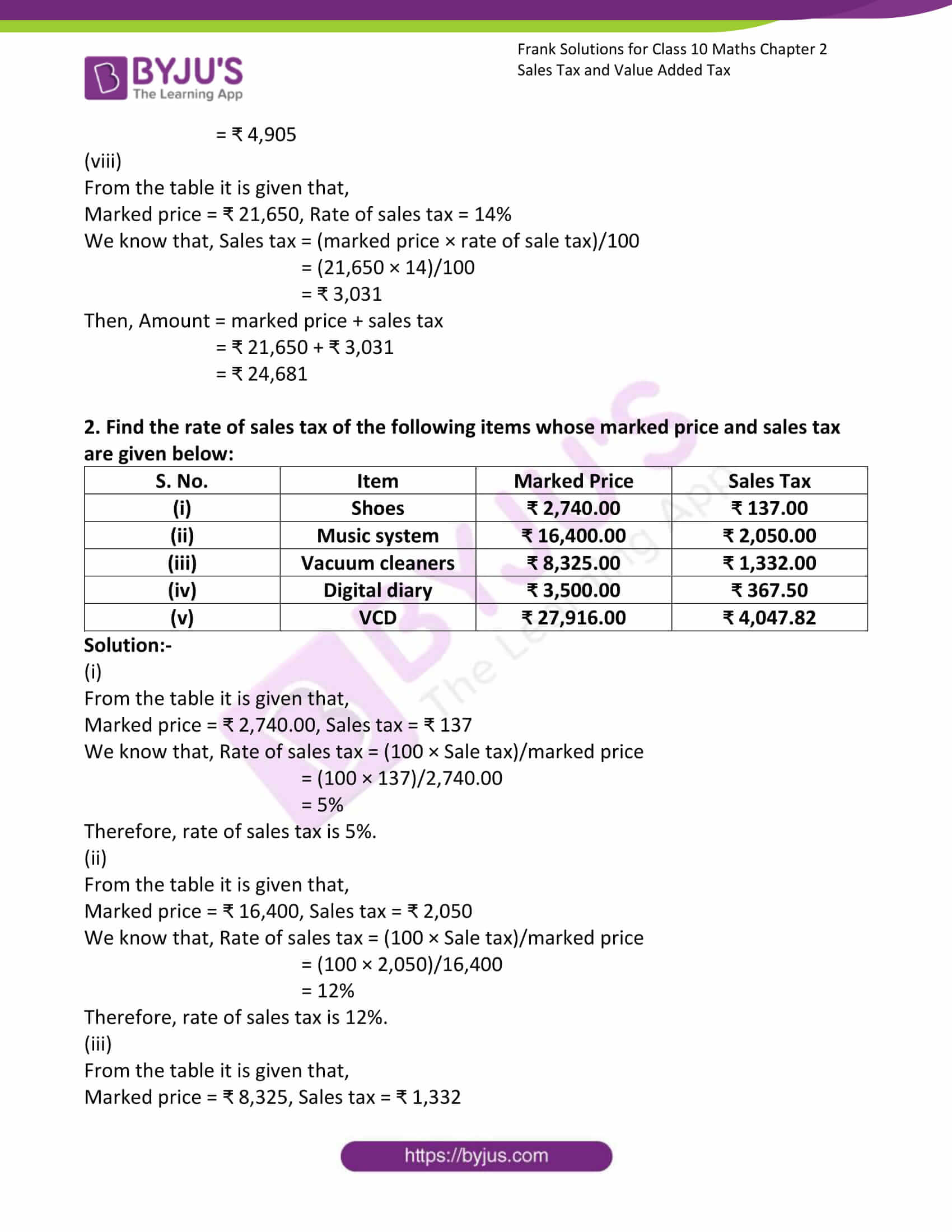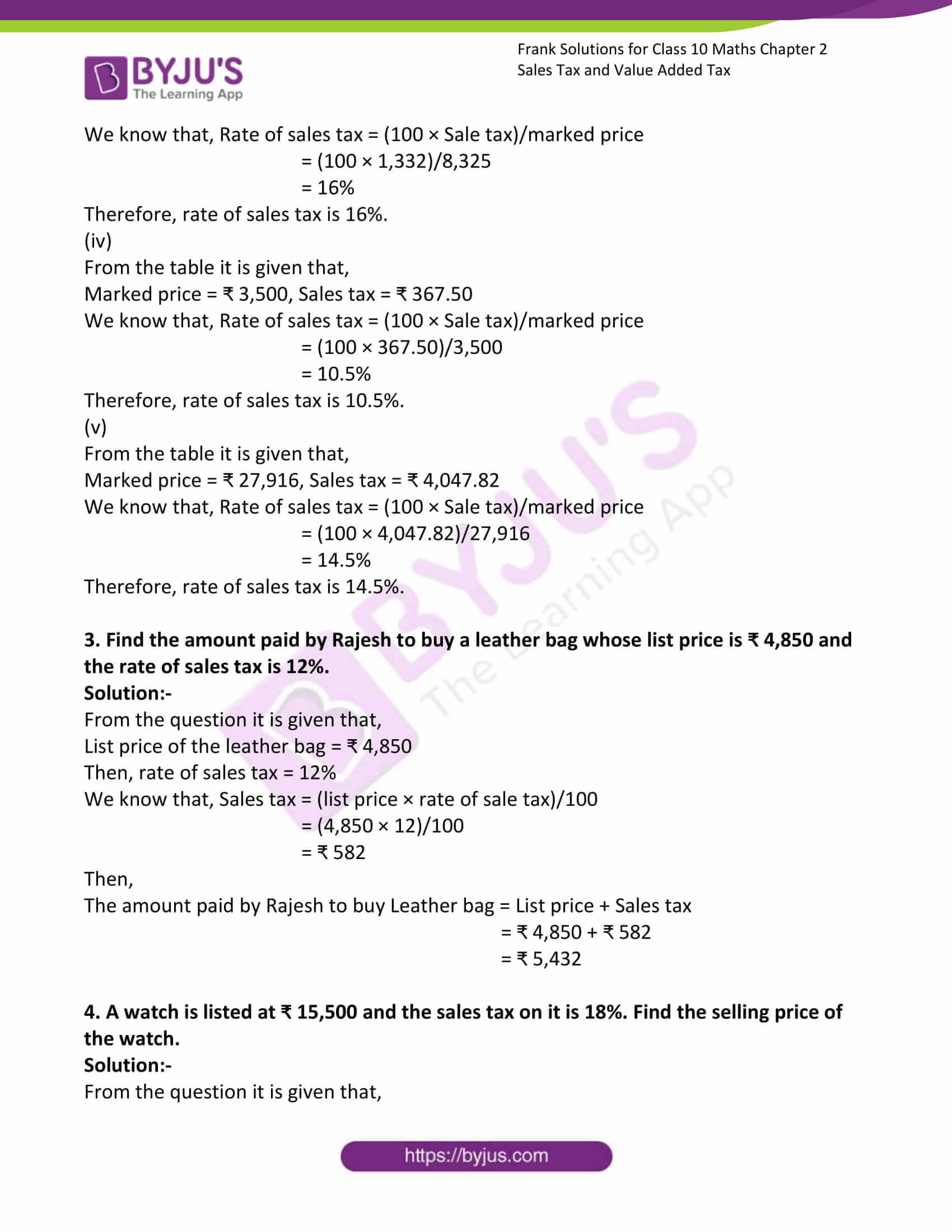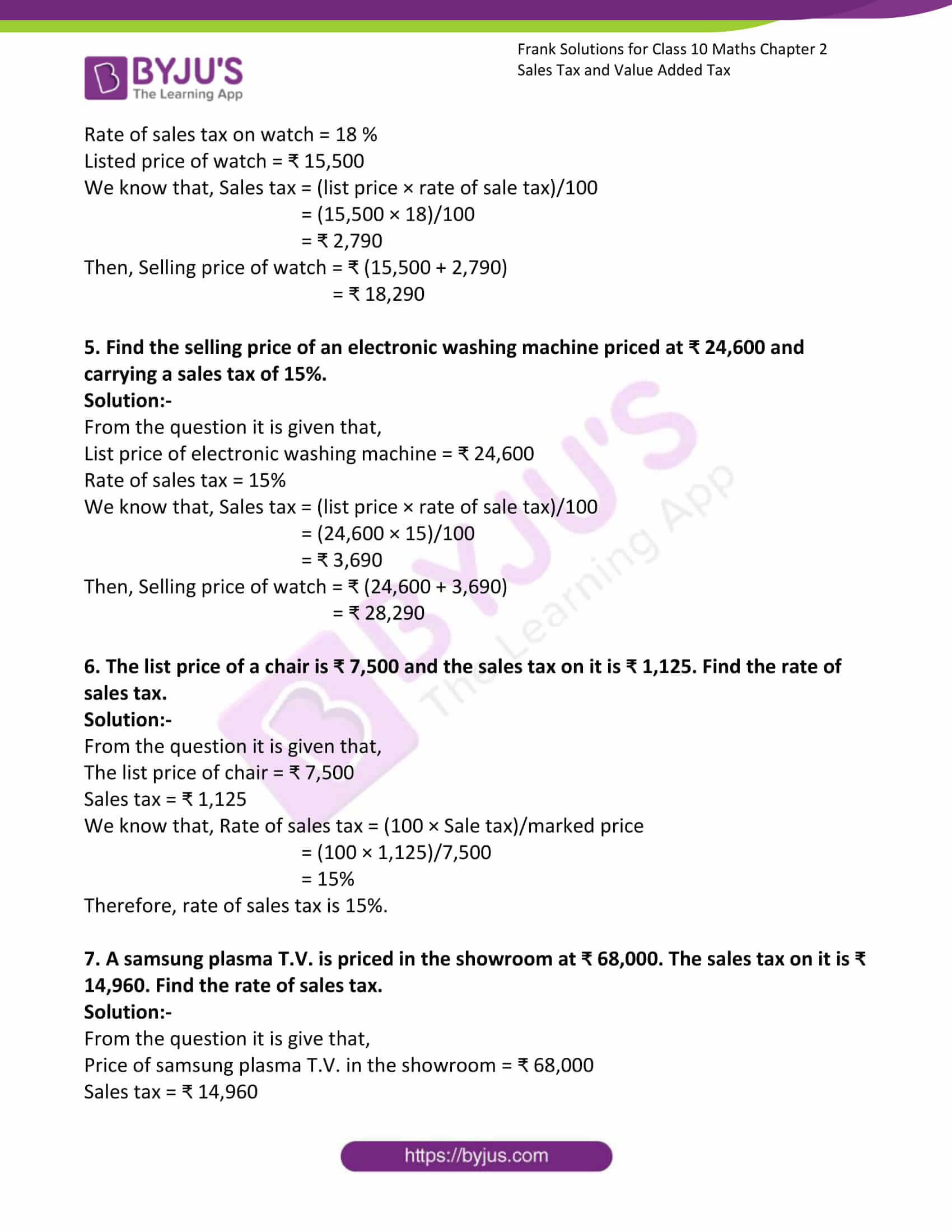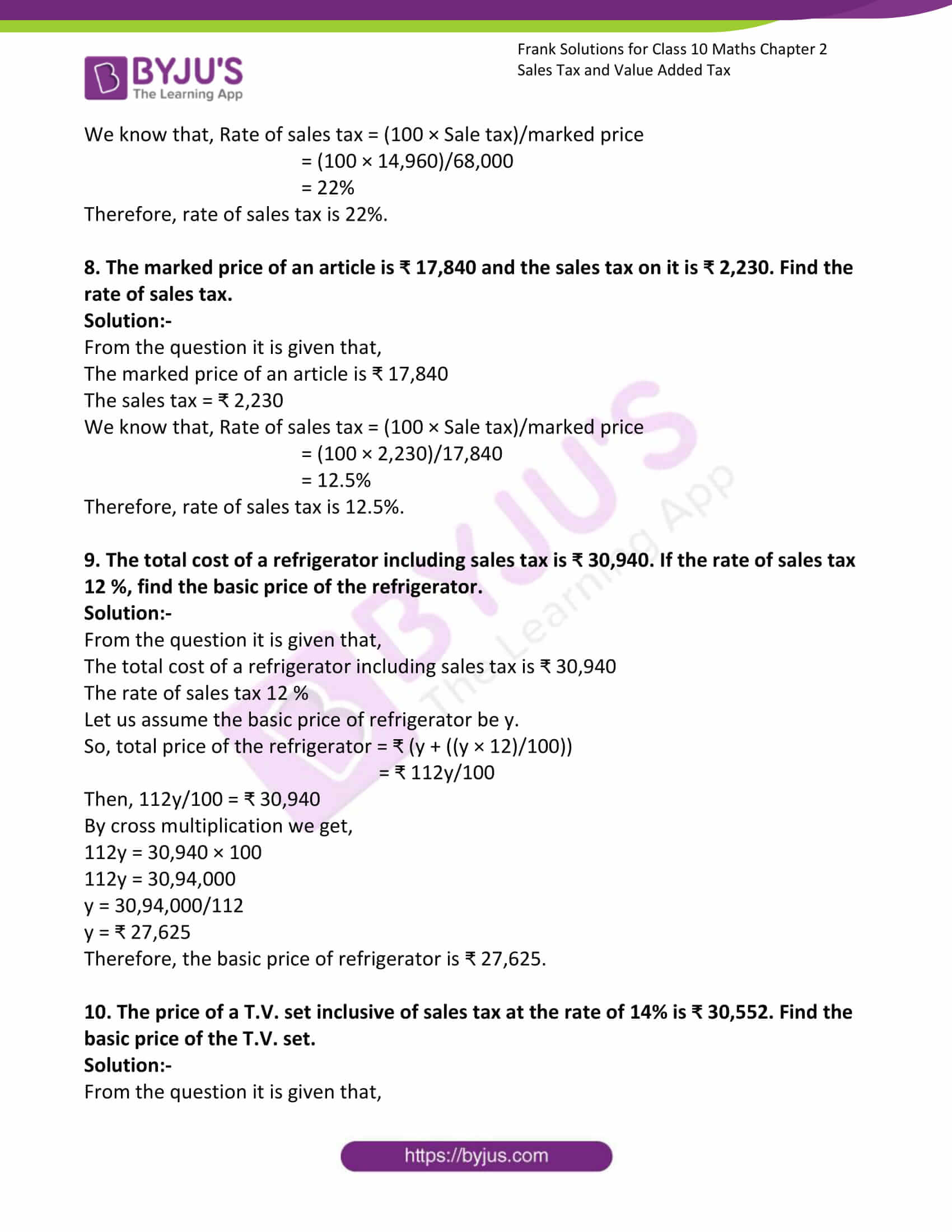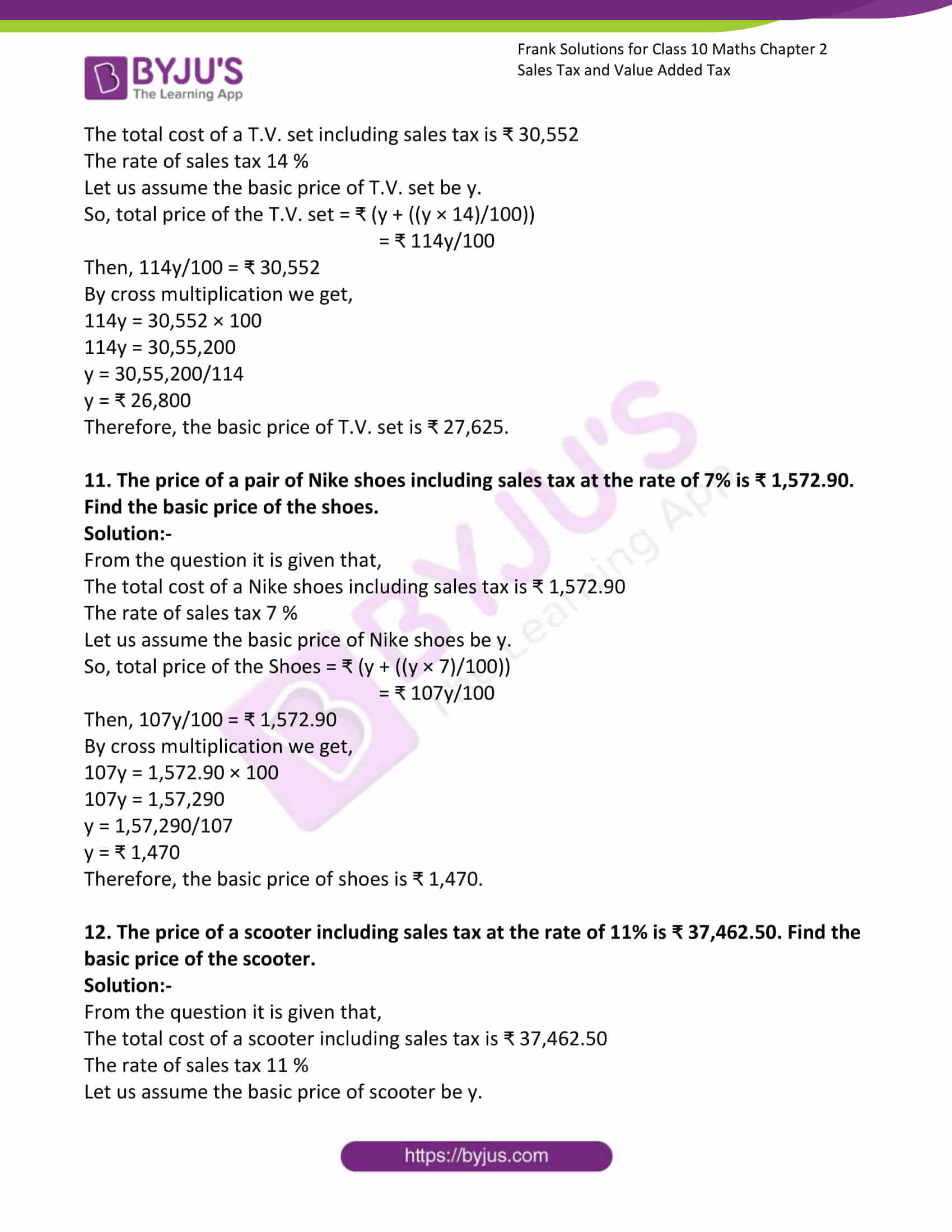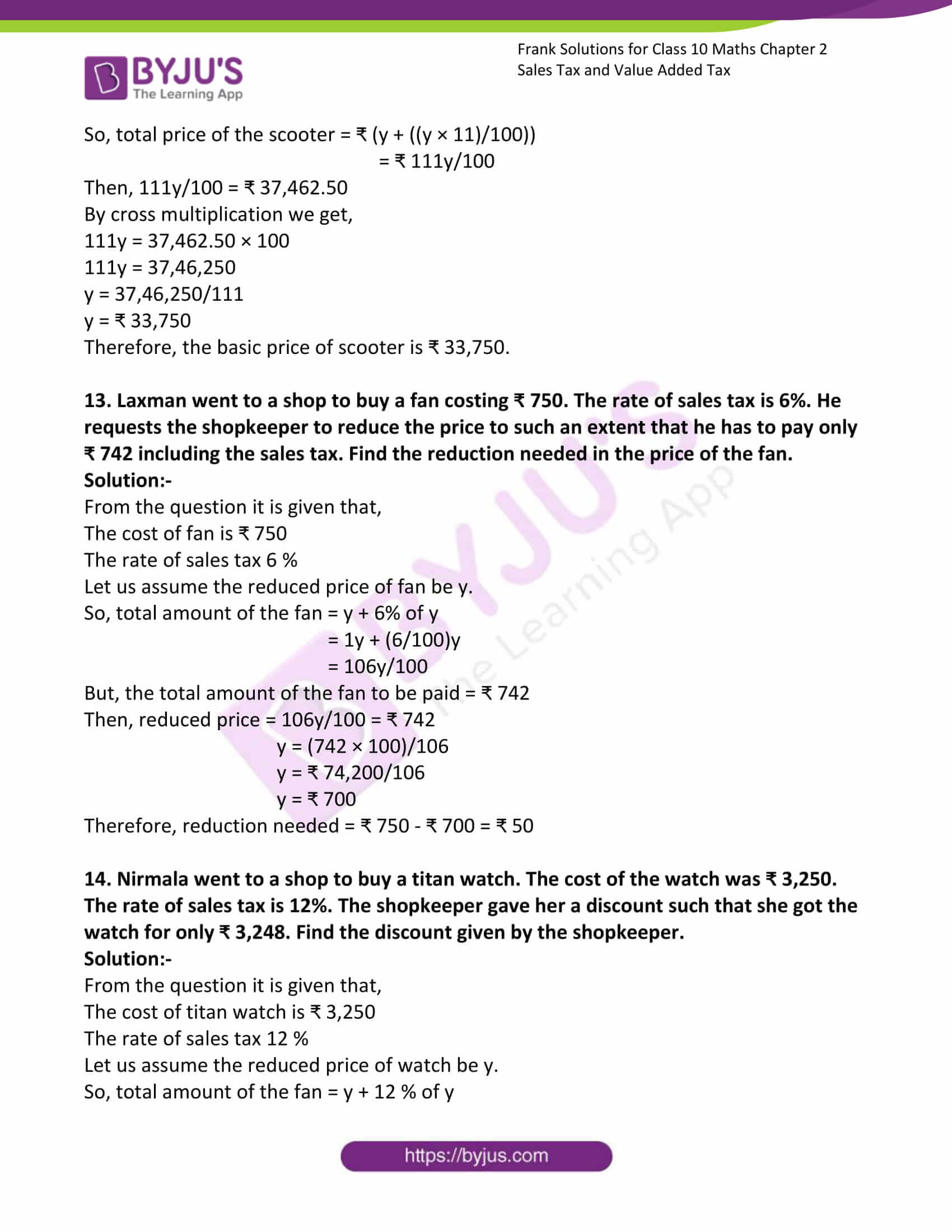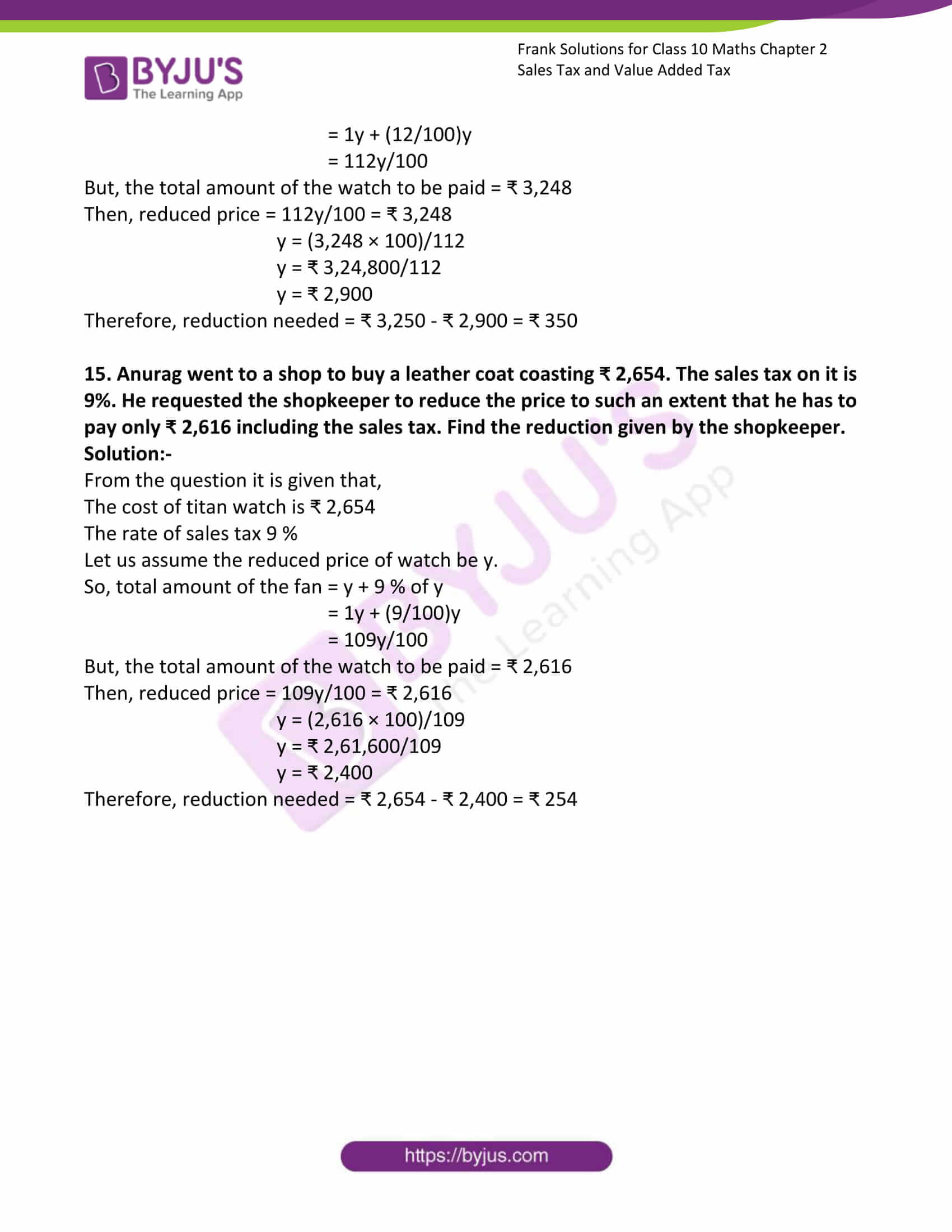### Access answers to Frank Solutions for Class 10 Maths Chapter 2 Sales Tax and Value Added Tax

1. The marked price and the rate of sales tax of different items are given below. Calculate the amount to be paid for each of them:

 S. No. Item Marked Price Rate of Sales Tax (i) Walkman ₹ 1,750.00 8.5% (ii) Washing machine ₹14,840.00 7.5% (iii) Computer ₹ 32,725.00 12% (iv) Sofa set ₹ 16,000.00 11.5% (v) T.V. ₹ 28,975.00 16% (vi) Jacket ₹ 1,260.00 10% (vii) Camera ₹ 4,500.00 9% (viii) Air conditioner ₹ 21,650.00 14%

Solution:-

(i)

From the table it is given that,

Marked price = ₹ 1,750, Rate of sales tax = 8.5%

We know that, Sales tax = (marked price × rate of sale tax)/100

= (1,750 × 8.5)/100

= ₹ 148.75

Then, Amount = marked price + sales tax

= ₹ 1,750 + ₹ 148.75

= ₹ 1,898.75

(ii)

From the table it is given that,

Marked price = ₹ 14,840, Rate of sales tax = 7.5%

We know that, Sales tax = (marked price × rate of sale tax)/100

= (14,840 × 7.5)/100

= ₹ 1,113

Then, Amount = marked price + sales tax

= ₹ 14,840 + ₹ 1,113

= ₹ 15,953

(iii)

From the table it is given that,

Marked price = ₹ 32,725, Rate of sales tax = 12%

We know that, Sales tax = (marked price × rate of sale tax)/100

= (32,725 × 12)/100

= ₹ 3,927

Then, Amount = marked price + sales tax

= ₹ 32,725 + ₹ 3,927

= ₹ 36,652

(iv)

From the table it is given that,

Marked price = ₹ 16,000, Rate of sales tax = 11.5%

We know that, Sales tax = (marked price × rate of sale tax)/100

= (16,000 × 11.5)/100

= ₹ 1,840

Then, Amount = marked price + sales tax

= ₹ 16,000 + ₹ 1,840

= ₹ 17,840

(v)

From the table it is given that,

Marked price = ₹ 28,975, Rate of sales tax = 16%

We know that, Sales tax = (marked price × rate of sale tax)/100

= (28,975 × 16)/100

= ₹ 4,636

Then, Amount = marked price + sales tax

= ₹ 28,975 + ₹ 4,636

= ₹ 33,611

(vi)

From the table it is given that,

Marked price = ₹ 1,260, Rate of sales tax = 10%

We know that, Sales tax = (marked price × rate of sale tax)/100

= (1,260 × 10)/100

= ₹ 126

Then, Amount = marked price + sales tax

= ₹ 1,260 + ₹ 126

= ₹ 1,385

(vii)

From the table it is given that,

Marked price = ₹ 4,500, Rate of sales tax = 9%

We know that, Sales tax = (marked price × rate of sale tax)/100

= (4,500 × 9)/100

= ₹ 405

Then, Amount = marked price + sales tax

= ₹ 4,500 + ₹ 405

= ₹ 4,905

(viii)

From the table it is given that,

Marked price = ₹ 21,650, Rate of sales tax = 14%

We know that, Sales tax = (marked price × rate of sale tax)/100

= (21,650 × 14)/100

= ₹ 3,031

Then, Amount = marked price + sales tax

= ₹ 21,650 + ₹ 3,031

= ₹ 24,681

2. Find the rate of sales tax of the following items whose marked price and sales tax are given below:

 S. No. Item Marked Price Sales Tax (i) Shoes ₹ 2,740.00 ₹ 137.00 (ii) Music system ₹ 16,400.00 ₹ 2,050.00 (iii) Vacuum cleaners ₹ 8,325.00 ₹ 1,332.00 (iv) Digital diary ₹ 3,500.00 ₹ 367.50 (v) VCD ₹ 27,916.00 ₹ 4,047.82

Solution:-

(i)

From the table it is given that,

Marked price = ₹ 2,740.00, Sales tax = ₹ 137

We know that, Rate of sales tax = (100 × Sale tax)/marked price

= (100 × 137)/2,740.00

= 5%

Therefore, rate of sales tax is 5%.

(ii)

From the table it is given that,

Marked price = ₹ 16,400, Sales tax = ₹ 2,050

We know that, Rate of sales tax = (100 × Sale tax)/marked price

= (100 × 2,050)/16,400

= 12%

Therefore, rate of sales tax is 12%.

(iii)

From the table it is given that,

Marked price = ₹ 8,325, Sales tax = ₹ 1,332

We know that, Rate of sales tax = (100 × Sale tax)/marked price

= (100 × 1,332)/8,325

= 16%

Therefore, rate of sales tax is 16%.

(iv)

From the table it is given that,

Marked price = ₹ 3,500, Sales tax = ₹ 367.50

We know that, Rate of sales tax = (100 × Sale tax)/marked price

= (100 × 367.50)/3,500

= 10.5%

Therefore, rate of sales tax is 10.5%.

(v)

From the table it is given that,

Marked price = ₹ 27,916, Sales tax = ₹ 4,047.82

We know that, Rate of sales tax = (100 × Sale tax)/marked price

= (100 × 4,047.82)/27,916

= 14.5%

Therefore, rate of sales tax is 14.5%.

3. Find the amount paid by Rajesh to buy a leather bag whose list price is ₹ 4,850 and the rate of sales tax is 12%.

Solution:-

From the question it is given that,

List price of the leather bag = ₹ 4,850

Then, rate of sales tax = 12%

We know that, Sales tax = (list price × rate of sale tax)/100

= (4,850 × 12)/100

= ₹ 582

Then,

The amount paid by Rajesh to buy Leather bag = List price + Sales tax

= ₹ 4,850 + ₹ 582

= ₹ 5,432

4. A watch is listed at ₹ 15,500 and the sales tax on it is 18%. Find the selling price of the watch.

Solution:-

From the question it is given that,

Rate of sales tax on watch = 18 %

Listed price of watch = ₹ 15,500

We know that, Sales tax = (list price × rate of sale tax)/100

= (15,500 × 18)/100

= ₹ 2,790

Then, Selling price of watch = ₹ (15,500 + 2,790)

= ₹ 18,290

5. Find the selling price of an electronic washing machine priced at ₹ 24,600 and carrying a sales tax of 15%.

Solution:-

From the question it is given that,

List price of electronic washing machine = ₹ 24,600

Rate of sales tax = 15%

We know that, Sales tax = (list price × rate of sale tax)/100

= (24,600 × 15)/100

= ₹ 3,690

Then, Selling price of watch = ₹ (24,600 + 3,690)

= ₹ 28,290

6. The list price of a chair is ₹ 7,500 and the sales tax on it is ₹ 1,125. Find the rate of sales tax.

Solution:-

From the question it is given that,

The list price of chair = ₹ 7,500

Sales tax = ₹ 1,125

We know that, Rate of sales tax = (100 × Sale tax)/marked price

= (100 × 1,125)/7,500

= 15%

Therefore, rate of sales tax is 15%.

7. A samsung plasma T.V. is priced in the showroom at ₹ 68,000. The sales tax on it is ₹ 14,960. Find the rate of sales tax.

Solution:-

From the question it is give that,

Price of samsung plasma T.V. in the showroom = ₹ 68,000

Sales tax = ₹ 14,960

We know that, Rate of sales tax = (100 × Sale tax)/marked price

= (100 × 14,960)/68,000

= 22%

Therefore, rate of sales tax is 22%.

8. The marked price of an article is ₹ 17,840 and the sales tax on it is ₹ 2,230. Find the rate of sales tax.

Solution:-

From the question it is given that,

The marked price of an article is ₹ 17,840

The sales tax = ₹ 2,230

We know that, Rate of sales tax = (100 × Sale tax)/marked price

= (100 × 2,230)/17,840

= 12.5%

Therefore, rate of sales tax is 12.5%.

9. The total cost of a refrigerator including sales tax is ₹ 30,940. If the rate of sales tax 12 %, find the basic price of the refrigerator.

Solution:-

From the question it is given that,

The total cost of a refrigerator including sales tax is ₹ 30,940

The rate of sales tax 12 %

Let us assume the basic price of refrigerator be y.

So, total price of the refrigerator = ₹ (y + ((y × 12)/100))

= ₹ 112y/100

Then, 112y/100 = ₹ 30,940

By cross multiplication we get,

112y = 30,940 × 100

112y = 30,94,000

y = 30,94,000/112

y = ₹ 27,625

Therefore, the basic price of refrigerator is ₹ 27,625.

10. The price of a T.V. set inclusive of sales tax at the rate of 14% is ₹ 30,552. Find the basic price of the T.V. set.

Solution:-

From the question it is given that,

The total cost of a T.V. set including sales tax is ₹ 30,552

The rate of sales tax 14 %

Let us assume the basic price of T.V. set be y.

So, total price of the T.V. set = ₹ (y + ((y × 14)/100))

= ₹ 114y/100

Then, 114y/100 = ₹ 30,552

By cross multiplication we get,

114y = 30,552 × 100

114y = 30,55,200

y = 30,55,200/114

y = ₹ 26,800

Therefore, the basic price of T.V. set is ₹ 27,625.

11. The price of a pair of Nike shoes including sales tax at the rate of 7% is ₹ 1,572.90. Find the basic price of the shoes.

Solution:-

From the question it is given that,

The total cost of a Nike shoes including sales tax is ₹ 1,572.90

The rate of sales tax 7 %

Let us assume the basic price of Nike shoes be y.

So, total price of the Shoes = ₹ (y + ((y × 7)/100))

= ₹ 107y/100

Then, 107y/100 = ₹ 1,572.90

By cross multiplication we get,

107y = 1,572.90 × 100

107y = 1,57,290

y = 1,57,290/107

y = ₹ 1,470

Therefore, the basic price of shoes is ₹ 1,470.

12. The price of a scooter including sales tax at the rate of 11% is ₹ 37,462.50. Find the basic price of the scooter.

Solution:-

From the question it is given that,

The total cost of a scooter including sales tax is ₹ 37,462.50

The rate of sales tax 11 %

Let us assume the basic price of scooter be y.

So, total price of the scooter = ₹ (y + ((y × 11)/100))

= ₹ 111y/100

Then, 111y/100 = ₹ 37,462.50

By cross multiplication we get,

111y = 37,462.50 × 100

111y = 37,46,250

y = 37,46,250/111

y = ₹ 33,750

Therefore, the basic price of scooter is ₹ 33,750.

13. Laxman went to a shop to buy a fan costing ₹ 750. The rate of sales tax is 6%. He requests the shopkeeper to reduce the price to such an extent that he has to pay only ₹ 742 including the sales tax. Find the reduction needed in the price of the fan.

Solution:-

From the question it is given that,

The cost of fan is ₹ 750

The rate of sales tax 6 %

Let us assume the reduced price of fan be y.

So, total amount of the fan = y + 6% of y

= 1y + (6/100)y

= 106y/100

But, the total amount of the fan to be paid = ₹ 742

Then, reduced price = 106y/100 = ₹ 742

y = (742 × 100)/106

y = ₹ 74,200/106

y = ₹ 700

Therefore, reduction needed = ₹ 750 – ₹ 700 = ₹ 50

14. Nirmala went to a shop to buy a titan watch. The cost of the watch was ₹ 3,250. The rate of sales tax is 12%. The shopkeeper gave her a discount such that she got the watch for only ₹ 3,248. Find the discount given by the shopkeeper.

Solution:-

From the question it is given that,

The cost of titan watch is ₹ 3,250

The rate of sales tax 12 %

Let us assume the reduced price of watch be y.

So, total amount of the fan = y + 12 % of y

= 1y + (12/100)y

= 112y/100

But, the total amount of the watch to be paid = ₹ 3,248

Then, reduced price = 112y/100 = ₹ 3,248

y = (3,248 × 100)/112

y = ₹ 3,24,800/112

y = ₹ 2,900

Therefore, reduction needed = ₹ 3,250 – ₹ 2,900 = ₹ 350

15. Anurag went to a shop to buy a leather coat coasting ₹ 2,654. The sales tax on it is 9%. He requested the shopkeeper to reduce the price to such an extent that he has to pay only ₹ 2,616 including the sales tax. Find the reduction given by the shopkeeper.

Solution:-

From the question it is given that,

The cost of titan watch is ₹ 2,654

The rate of sales tax 9 %

Let us assume the reduced price of watch be y.

So, total amount of the fan = y + 9 % of y

= 1y + (9/100)y

= 109y/100

But, the total amount of the watch to be paid = ₹ 2,616

Then, reduced price = 109y/100 = ₹ 2,616

y = (2,616 × 100)/109

y = ₹ 2,61,600/109

y = ₹ 2,400

Therefore, reduction needed = ₹ 2,654 – ₹ 2,400 = ₹ 254